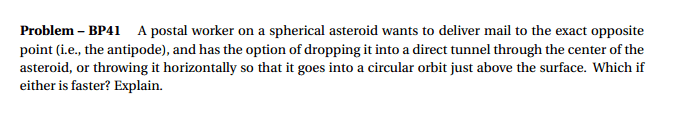# Period of falling through asteroid vs orbit

## Homework StatementSee below

## The Attempt at a Solution

My book derives the period of oscillation through the tunnel:
T = 2pi/w = 2pi*sqrt(m/k) = 2pi*sqrt(3/(4pi*G*p)) = sqrt(3pi/(G*p))
Where p is the density of the asteroid, and G is the Newton's gravitational constant.

I know that the orbit velocity is found by equating the gravity force to the necessary centripetal force:
mg = mv^2/r
v = sqrt(r*g)
So the period is 2*pi*r/v = 2pi*sqrt(r/g)

I know these two periods are equal. Can anyone help me with putting them and similar terms and proving that they are?

## Answers and Replies

ehild
Homework Helper
What is g on that spherical asteroid? Do you see any connection between "g" and the Universal Law of Gravitation?

ehild

Last edited:
If you click on "Go advanced" you will find that it is easy to use the letter π instead of the word "pi".

Extra credit: what if the asteroid is differentiated, that is the density at its core is higher than at its mantle.